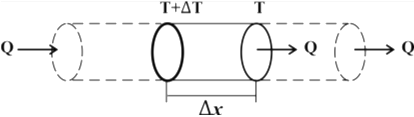# Thermal Conductivity

## Thermal conductivity definition

Thermal conductivity of a solid is a measure of the ability of the solid to conduct heat through it.
Greater is the thermal conductivity of a solid, the greater is its ability to conduct heat through it. If a solid has lower heat conductivity its ability to conduct heat through it would be lower.
The rate of heat conduction through a medium depends on following factors :-
• geometry of the medium,
• its thickness, and
• the material of the medium.
• It also depends on the temperature difference across the medium.

## Thermal Conductivity Derivation

In this section we would have a look at how we can derive Thermal Conductivity equation or Thermal conduction equation.
Let us consider a piece of material made in the form of a bar of thickness$\Delta x$ and area of cross-section $A$ as shown below in the figure.Consider this figure for the derivation of Thermal Conductivity equation where we have considered a piece of material made in the form of a bar of thickness$\Delta x$ and area of cross-section $A$ From this figure we can see that $\Delta x$ is the perpendicular distance between two isothermal plane surfaces. These two isothermal surfaces temperatures $(T+\Delta T)$ and $T$. Note that $(T+\Delta T) > T$.
Now the heat that flows perpendicular to the faces for a time $t$ is measured. The experiment for measuring heat is repeated with other bars of same material but with different values of $\Delta x$ and $A$. the results of such experiments show that, for a given value of$\Delta T$
1. The quantity of heat conducted is directly proportional to the area of each surface i.e., $Q\propto A$
2. Quantity of heat conducted is directly proportional to the temperature gradient in direction of heat flow. That is
$Q\propto -\frac{\Delta T}{\Delta x}$
Provided both $\Delta T$ and $\Delta x$ is small.
3. Quantity of heat conducted is directly proportional to the time t i.e., $Q\propto t$
Combining all together we get the relation \begin{align} & Q\propto -A\frac{\Delta T}{\Delta x}\cdot t \nonumber \\ & \Rightarrow Q=-KA\text{ }\frac{\Delta T}{\Delta x}\cdot t \\ \end{align} This relation is mathematical expression for the basic low of heat conduction and is known as Fourier’s law of heat conduction . It is also known as thermal conduction equation.
Here factor $K$ is the proportionality constant and is known coefficient thermal conductivity of the material. Its value depends on the nature of material used. Thermal conductivity is the property of the material and it is the ability of the substance to transfer heat.
If in above equation (1) we put
$A=1m^2$ , $\Delta x=1m$, $\Delta T=-1K$ and $t=1 \,sec$ then,
$Q=K$
The value of thermal conductivity determines the quantity of heat passing per unit area at a temperature drop of 1k per unit length in unit time.

### Special case for thermal conductivity equation :-

We know that The quantity Q/t is the time rate of heat flow (i.e., heat flow per second) from the hotter face to the colder face and is at right angles to the faces [Unit = W (watt)]. So, $$\frac{Q}{t}=-KA\text{ }\frac{\Delta T}{\Delta x}$$ If the area of cross-section is not uniform or if the steady state conditions are not reached, the equation can be applied to a thin layer of material normal to the direction of flow of heat. This could be a limiting case where $\Delta x\to 0$.
Due to this limiting case rate of heat flow that is given by
$H= \frac{dQ}{dt}=-KA\frac{dT}{dx}$
Where $dQ$ is the amount heat crossing the thin layer of thickness $dx$ in time $dT$. $dT$ is the temperature difference across the layer of thickness $dx$.

## Units of dimensions of coefficient of thermal conductivity

### Units of thermal conductivity

$\text{K=}\frac{Q\Delta x}{A(\Delta T)t}$
SI unit of $K$ is $=\frac{J.m}{{{m}^{2}}K\cdot s}=J{{s}^{-1}}{{m}^{-1}}=w{{m}^{-1}}{{k}^{-1}}$
CGS unit of $K$ is $Cal\cdot {{s}^{-1}}c{{m}^{-1}}^{0}{{C}^{-1}}$

### Dimensions of thermal conductivity

Dimensions of thermal conductivity $K$ are
$=\frac{[M{{L}^{2}}{{T}^{-2}}]\cdot [L]}{[{{L}^{2}}]\cdot [K]\cdot [T]}=[ML{{T}^{-3}}{{K}^{-1}}]$

## Solved problems on thermal conductivity

Question 1A slab of stone of area $0.36 m^2$ and thickness $0.1 m$ is exposed on the lower surface to steam at $100^{\circ}C$. A block of ice at $0^{\circ}C$ rests on the upper surface of the slab. If in one hour, $4.8 kg$ of ice is melted, calculate the thermal conductivity of the stone.
Answer 1The amount of heat flowing through the slab is
$$Q=\frac{kA(T_1 -T_2)t}{x}$$ According to the conditions of the problem, $Q = mL$.
$$mL=\frac{kA(T_1 -T_2)t}{x}$$ Here it is given that
$m= 4.8 \, kg$ ; $L = 80 \, kcal/kg$ ; $A = 0.36 \, m^2$; $T_1 - T_2= 100 - 0 = 100^{\circ}C$
Now $t=1 \, hour = 3600 \, s \, , \, x=0.1 \, m \, k=?$
Therefore, $$4.8 \times 80 = \frac{k \times 0.36 \times 100 \times 3600}{0.1}$$ Or, thermal conductivity $k=3 \times 10^{-4}\, kcal s^{-1}m{-1}^{\circ}C^{-1}$
QuestionTwo metal cubes A and B of the same size are arranged as shown in the figure. The extreme ends of the combination are maintained at the indicated temperatures. The arrangement is thermally insulated. The coefficient of thermal conductivity of $A$ and $B$ are $300 W/m ^{\circ}C$ and $200 W/m ^{\circ}C$, respectively. After steady state is reached , what will be the temperature $T$ of the interface? (IIT 1996)
AnswerIn the steady state, Rate of flow of heat through cube A = Rate of flow of heat through cube B
$$\frac{K_1 A(100-T)}{x}=\frac{K_2 A (T-0)}{x}$$ $$\frac{300 A(100-T)}{x}=\frac{200 A (T-0)}{x}$$ or,
$$300-3T=2T$$ or, $$5T=300$$ $$T=60^{\circ}C$$ Question The two ends of a rod of length $L$ and uniform cross-sectional area $A$ are kept at temperatures $T_1$ and $T_2$ where $(T_1 > T_2)$. The rate of heat transfer , $\frac{dQ}{dt}$ through the rod in a steady state is given by
(a) $\frac{dQ}{dt}=\frac{K(T_1 - T_2)}{LA}$
(b) $\frac{dQ}{dt}=KLA(T_1 - T_2)$
(c) $\frac{dQ}{dt}=\frac{KA(T_1 - T_2)}{L}$
(d) $\frac{dQ}{dt}=\frac{KL(T_1 - T_2)}{A}$